Courses

# Technical Test SSC JE: Electrical Engineering (EE)- 15

## 100 Questions MCQ Test Electrical Engineering SSC JE (Technical) | Technical Test SSC JE: Electrical Engineering (EE)- 15

Description
This mock test of Technical Test SSC JE: Electrical Engineering (EE)- 15 for Electrical Engineering (EE) helps you for every Electrical Engineering (EE) entrance exam. This contains 100 Multiple Choice Questions for Electrical Engineering (EE) Technical Test SSC JE: Electrical Engineering (EE)- 15 (mcq) to study with solutions a complete question bank. The solved questions answers in this Technical Test SSC JE: Electrical Engineering (EE)- 15 quiz give you a good mix of easy questions and tough questions. Electrical Engineering (EE) students definitely take this Technical Test SSC JE: Electrical Engineering (EE)- 15 exercise for a better result in the exam. You can find other Technical Test SSC JE: Electrical Engineering (EE)- 15 extra questions, long questions & short questions for Electrical Engineering (EE) on EduRev as well by searching above.
QUESTION: 1

Solution:
QUESTION: 2

Solution:
QUESTION: 3

### Hysteresis losses in a d.c. machine are proportional to

Solution:
QUESTION: 4

Torque/ weight ratio of an instrument indicates

Solution:
QUESTION: 5

Series reactors are used to

Solution:
QUESTION: 6

What is the range for a   3(1/2)digit digital voltmeter ?

Solution:
QUESTION: 7

........ is the region of a transistor which has highest conductivity.

Solution:
QUESTION: 8

Two coils have self-inductances of 0.09 H and 0.01 H and mutual inductance of 0.015 H. The coefficient of coupling between coils is

Solution:
QUESTION: 9

Two resistances R1 and R2 give combined resistance of 4.5 ohms when in series and 1 ohm when in parallel. The resistance are

Solution:
QUESTION: 10

In a star-connected system without neutral grounding, zero-sequence currents are

Solution:
QUESTION: 11

Sensitivity of a voltmeter is given as

Solution:
QUESTION: 12

If one of the central springs of a Permanent Magnet Moving Coil ammeter is broken then when connected it will read

Solution:
QUESTION: 13

The positive sequence current of a transmission line is :

Solution:
QUESTION: 14

The teeth in the armature of a dc machine are sometimes skewed. This is done to reduce

Solution:
QUESTION: 15

When a line-to-ground fault occurs, the current in the faulted phase is 100 A. The zero-sequence current in this case will be :

Solution:
QUESTION: 16

Resistance across A and B in the circuit shown below is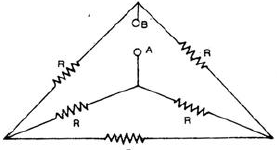Solution:
QUESTION: 17

In a CRT the highest positive potential is given to

Solution:
QUESTION: 18

the purpose of the synchronising control in a CRO is to

Solution:
QUESTION: 19

Power factor of an inductive circuit can be improved by connecting a capacitor to it in

Solution:
QUESTION: 20

In measurements made using a Q-meter impedance elements should preferably be connected in

Solution:
QUESTION: 21

Wagner Eearth devices in ac bridge circuits are used for

Solution:

Wagner’s earth devices are used in ac bridge circuits to eliminate the effect of earth capacitance.

QUESTION: 22

When the supply terminals of a dc shunt motor are interchanged

Solution:
QUESTION: 23

The current of a single-phase load drawn from a 3-phase system has:

Solution:
QUESTION: 24

Systematic error are

Solution:
QUESTION: 25

The minimum number of wattmeter (s) required to measure 3-phase 3-wire balanced or unbalance power is

Solution:
QUESTION: 26

The voltage coil of a single phase house energy meter

Solution:
QUESTION: 27

Which one of the following methods decreases the error due to connections in a dynamometer type wattmeter ?

Solution:
QUESTION: 28

If the positive-, negative-and zero-sequence reactances of an element of a power system are 0.3, 0.3 and 0.8 pu respectively, then the element would be a

Solution:
QUESTION: 29

A separately excited dc generator is feeding a dc shunt motor. If the load torque on the motor is halved approximately

Solution:
QUESTION: 30

A manganin swamp resistance is connected in series with a moving coil ammeter consisting of a milliameeter and a suitable shunt in order of

Solution:
QUESTION: 31

If starter is not used dc motor for starting, it will draw a starting current which

Solution:
QUESTION: 32

The deflecting torque of a moving iron instrument is

Solution:
QUESTION: 33

If all the sequence voltages at the fault point in a power system are equal, then the fault is a

Solution:
QUESTION: 34

A set of independent current measurements taken by four observers was recorded as : 117.02 mA, 117.11 mA, 117.08 mA and 117.03 mA. What is the range of error ?

Solution:
QUESTION: 35

Two coils having equal resistances but different inductances are connected in series. The time constant of the series combination is the

Solution:
QUESTION: 36

The zero-sequence circuit of the three-phase transformer shown in the figure is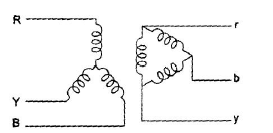Solution:
QUESTION: 37

The accuracy of Kelvin’s double bridge for the measurement of low resistance is high because the bridge

Solution:
QUESTION: 38

The octal equivalent of decimal 98 is

Solution:
QUESTION: 39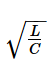has the dimension of

Solution:
QUESTION: 40

Steady state stability of a power system is improved by

Solution:
QUESTION: 41

The transistor is usually encapsuled in –

Solution:
QUESTION: 42

An alternating e.m.f. is applied to a pure inductance such that inductive reactance is 10 W.
If the frequency of a.c. is doubled, the reactance will become

Solution:
QUESTION: 43

The positive-sequence current for a L-L fault of a 2kV system is 1,400 A, and corresponding current for a L-L-G fault is 2,220 A.

The zero-sequence impedance of the system is

Solution:
QUESTION: 44

An RLC resonant circuit has a resonance frequency of 1.5 MHz and a bandwidth of 10
kHz. If C = 150 pF, then the effective resistance of the circuit will be

Solution:
QUESTION: 45

Match List I with List II and select the correct answer using the codes given below the lists: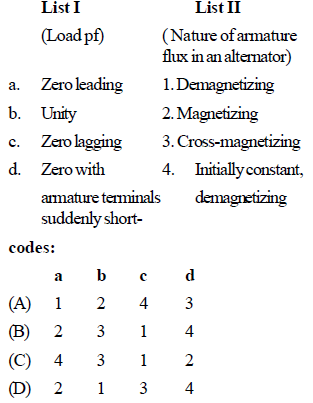Solution:
QUESTION: 46

For the same voltage drop, increasing the voltage of a distributor n-times.

Solution:
QUESTION: 47

A single – Phase, 200 V alternator has armature resistance and reactance of 0.8W and 4.94W respectively. The voltage regulation of the alternator at 100 A load at 0.8 leading power factor is

Solution:
QUESTION: 48

The insulation coordination for UHV lines (above 500 kV) is done base on

Solution:
QUESTION: 49

A SCIM having a rated slip of 4% on full load has a starting torque the same as full-load torque.The starting current is

Solution:
QUESTION: 50

Lightning is a huge spark caused by electrical discharge taking place between

Solution:
QUESTION: 51

A network N ' is dual of network N if

Solution:
QUESTION: 52

Which of the following is usually not the generating voltage ?

Solution:
QUESTION: 53

Post-deflection is required in CRO, if input signal frequency is

Solution:
QUESTION: 54

In a resistance loaded, RC coupled amplifier the D.C. component is blocked by –

Solution:
QUESTION: 55

An higher scale ammeter is used to measure too low current. The measurement would have low

Solution:
QUESTION: 56

Dominating harmonics in IM is

Solution:
QUESTION: 57

A 3-Phase star-connected symmetrical load consumes P watts of power from a balance supply. If the same load is connected in delta to the same supply , the power consumption will be

Solution:
QUESTION: 58

Dead zone of an instrument is

Solution:
QUESTION: 59

Which of the following components of a D.C. generator plays vital role for providing direct current of a D.C. generator ?

Solution:
QUESTION: 60

What is the slope of the line due to1/jW  factor in magnitude part of Bode plot?

Solution:
QUESTION: 61

For the circuit shown in fig. the meter will read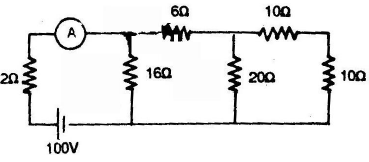Solution:
QUESTION: 62

The ratio of starting torque to full load torque is least in case of—

Solution:
QUESTION: 63

An indicating instrument is more sensitive if its torque to weight ratio is

Solution:
QUESTION: 64

When a capacitor was connected to the terminal of an ohmmeter the pointer indicated a low resistance initially and then slowly came to infinity position. This shows that the capacitor is

Solution:
QUESTION: 65

Impulse ratios of insulators and lightning arresters should be

Solution:
QUESTION: 66

For complete protection of a 3-phase transmission line we require

Solution:
QUESTION: 67

Hopkinson’s test is conducted at—

Solution:
QUESTION: 68

The difference between the indicated value and the true value of a quantity is

Solution:
QUESTION: 69

Which of the following is the fastest switching device?

Solution:
QUESTION: 70

Consider the protection system shown in the figure. The circuit breakers numbered from 1 to
7 are of identical type. A single line to ground fault with zero fault impedance occurs at the
midpoint of the line (at point F), but circuit breaker 4 fails to operate ("stuck breaker"). If the relays
are coordinated correctly, a valid sequence of circuit breaker operation is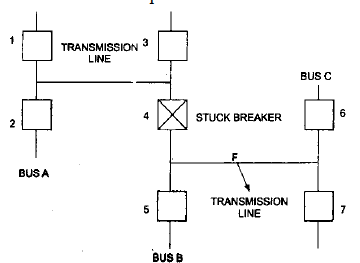Solution:
QUESTION: 71

In an Anderson bridge, the unknown inductance is measured in term of known

Solution:
QUESTION: 72

Mho relay is usually employed for the protection of

Solution:
QUESTION: 73

To measure 5 volts. If one selects a 0 – 100 V range voltmeter which is accurate within + 1%,then the error in this measurement may be up to

Solution:
QUESTION: 74

The full-load copper loss and iron loss of a transformer are 6,400 W and 5,000 W respectively. The copper loss and iron loss at half load will be, respectively

Solution:
QUESTION: 75

Curve A and B represent the properties for materials which have

Solution:
QUESTION: 76

Systematic error are

Solution:
QUESTION: 77

Vibration galvanometers, tuneable amplifiers and head phones are used in

Solution:
QUESTION: 78

An impedance relay is a

Solution:
QUESTION: 79

If the readings of the two wattmeters are equal and positive in two wattmeter method, the load pf in a balanced 3-phase 3-wire circuit will be

Solution:
QUESTION: 80

The most serious consequence of a major uncleared short-circuit fault could be

Solution:
QUESTION: 81

A distribution transformer is selected on the basis of

Solution:
QUESTION: 82

Under the condition of resonance in R-L-C series circuit, the pf of the circuit is

Solution:
QUESTION: 83

Consider the following statements associated with an energy meter.
1. It is an integrating type instrument.
2. It is an induction type instrument.
3. It uses a permanent magnet for rotation of aluminium disc.
4. it employs a high control torque.
Which of these statements are correct ?

Solution:
QUESTION: 84

In a.c. circuit, the power is consumed only in

Solution:
QUESTION: 85

Which portion of the transmission system is more prone to faults ?

Solution:
QUESTION: 86

An ideal dc generator has a regulation of ____ per cent.

Solution:
QUESTION: 87

The maximum power that can be distributed in the load in the circuit shown in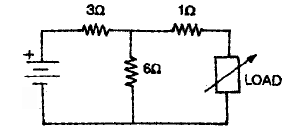Solution:
QUESTION: 88

Which one of the following is the main cause of creeping in the induction type energy meters ?

Solution:
QUESTION: 89

The errors introduced by an instrument fall in which category ?

Solution:
QUESTION: 90

Which portion of the power system is least prone to faults ?

Solution:
QUESTION: 91

At resonant frequency an R-L-C circuit draws maximum current due to the reason that

Solution:
QUESTION: 92

Schering bridge can be used to measure which one of the following ?

Solution:
QUESTION: 93

If an induction type energy meter runs fast, it can be slowed down by

Solution:
QUESTION: 94

The teeth in the armature of a dc machine are sometimes skewed. This is done to reduce

Solution:
QUESTION: 95

The maximum short-circuit current occurs in the case of:

Solution:
QUESTION: 96

The capacitance and loss angle of a given capacitor specimen are best measured by

Solution:
QUESTION: 97

Impedance relay may be

Solution:
QUESTION: 98

The most commonly used input signal (s) in control system is/are

Solution:
QUESTION: 99

Wattless current is possible, only in

Solution:
QUESTION: 100

For a fault at the terminals of synchronous generator, the fault current is maximum for a

Solution: